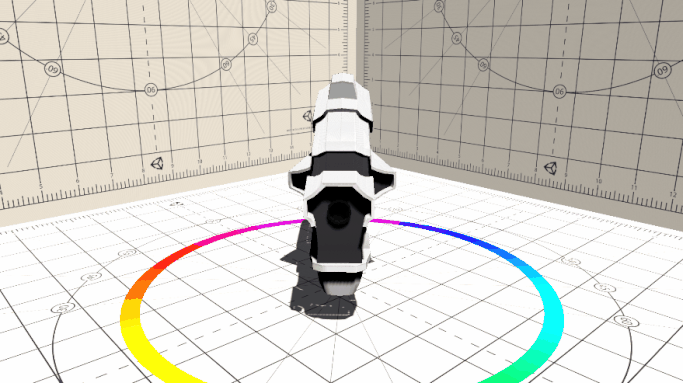The normal scene without any effects.

## The Basics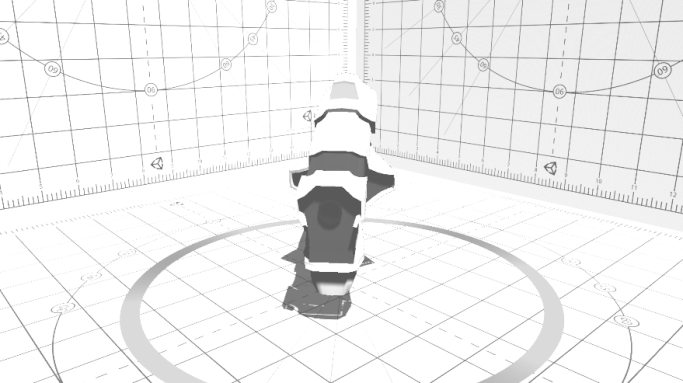Here you can see the scene with basic changes (bright­ness, con­trast and gray­scale).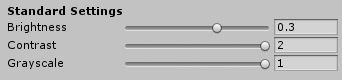Code:

//Get the colors of the screen.
fixed4 col = tex2D(_MainTex, i.uv);

//Calculate the average.
fixed greyValue = (col.r + col.g + col.b)/3;

//Create a color (fixed4) from this value.
fixed4 greyColor = fixed4(greyValue, greyValue, greyValue, 1);

//Lerp between normal and desaturated values (Slider from 0 to 1)
col = lerp(col, greyColor, _Saturation);Using two colors to create a gradient map. Darker values will be red and lighter values turn yellow.Code:

//Get the colors of the screen.
fixed4 col = tex2D(_MainTex, i.uv);

//Average value
fixed greyValue = (col.r + col.g + col.b)/3;
//Create Color from Average.
fixed4 greyColor = fixed4(greyValue, greyValue, greyValue, 1);

/*Create a new Color that lerps between the first and second
Gradient color according to the grayscale-value.*/

fixed4 gmColor = lerp(_GMColorOne, _GMColorTwo, greyValue);

col = lerp(col, gmColor, _GMIntensity);Same process as before using an image. More colorvalues can be stored that way (100% Black and White stay that way).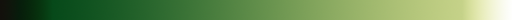Code:

//Get the colors of the screen.
fixed4 col = tex2D(_MainTex, i.uv);

//Average value
fixed greyValue = (col.r + col.g + col.b)/3;
//Create Color from Average.
fixed4 greyColor = fixed4(greyValue, greyValue, greyValue, 1);

/*Take a color from the image according to the brightness
fixed4 gmColor = tex2D(_GradMap, fixed2(greyValue, 0.5));

col = lerp(col, gmColor, _GMIntensity);

## Chromatic Aberration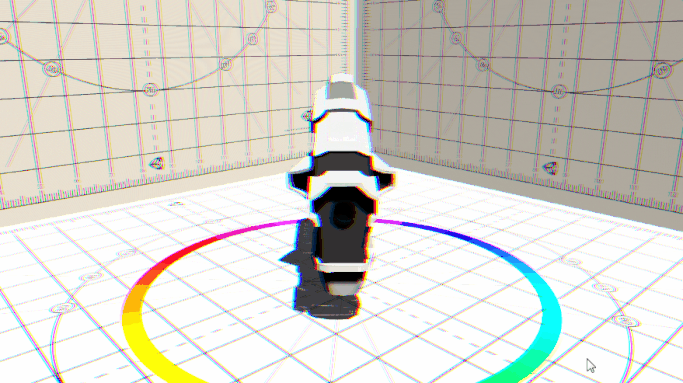Chromatic Aberration, often used to fake a camera lens.

Code:

/*Get RGB Values (including offset for chromatic aberration and offset for noiseMaps)*/

fixed4 colG = tex2D(_MainTex, fixed2(i.uv.x, i.uv.y));

fixed4 colR = tex2D(_MainTex, fixed2(i.uv.x + _CAoffset, i.uv.y));

fixed4 colB = tex2D(_MainTex, fixed2(i.uv.x - _CAoffset, i.uv.y));

//Set new Color
fixed4 col = fixed4(colR.r, colG.g, colB.b, 1);

## Distortion with Normal Maps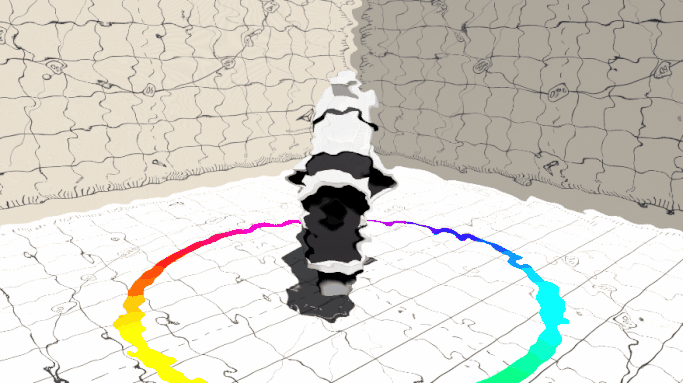The RGB Values of a Normal Map move the pixels of the screen.

This is the Normal Map I have used for this shader:Code:

// Get colors values from Noise Map*/
fixed2 rgNoise = tex2D(_NoiseMap, i.uv);

/*Get Color values from screen with the offset from noiseMaps*/
fixed4 colG = tex2D(_MainTex,
fixed2(i.uv.x + (rgNoise.r - rgNoise.g) * _NoiseIntens,
i.uv.y + (rgNoise.r - rgNoise.g) * _NoiseIntens));
fixed4 colR = tex2D(_MainTex,
fixed2(i.uv.x + (rgNoise.r - rgNoise.g) * _NoiseIntens,
i.uv.y + (rgNoise.r - rgNoise.g) * _NoiseIntens));
fixed4 colB = tex2D(_MainTex,
fixed2(i.uv.x + (rgNoise.r - rgNoise.g) * _NoiseIntens,
i.uv.y + (rgNoise.r - rgNoise.g) * _NoiseIntens));

//Set new Color
fixed4 col = fixed4(colR.r, colG.g, colB.b, 1);